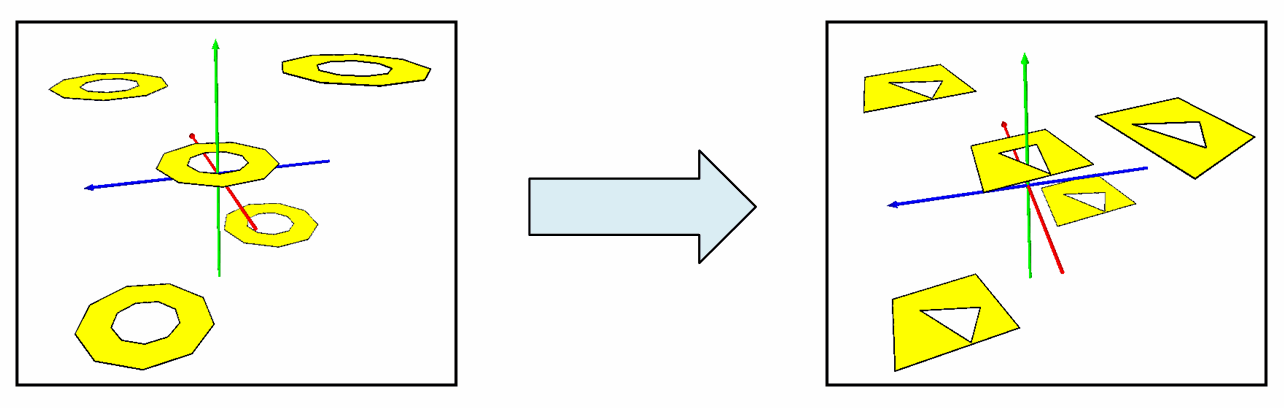This document is archived and information here might be outdated.  Recommended version.

IPolycurve3D.Generalize3D Method (ArcObjects .NET 10.7 SDK)
 ArcObjects Help for .NET developers > ArcObjects Help for .NET developers > ArcObjects namespaces > Geometry > ESRI.ArcGIS.Geometry > Interfaces > IP > IPolycurve3D Interface > IPolycurve3D.Generalize3D Method
 ArcGIS Developer Help

# IPolycurve3D.Generalize3D Method

Generalizes this polycurve using the Douglas-Peucker algorithm.

```[Visual Basic .NET]
Public Sub Generalize3D ( _
ByVal maxAllowableOffset As Double _
)
```
```[C#]
public void Generalize3D (
double maxAllowableOffset
);
```
```[C++]
HRESULT Generalize3D(
double maxAllowableOffset
);
```
```[C++]
Parameters
maxAllowableOffset
maxAllowableOffset is a parameter of type double
```

#### Product Availability

Available with ArcGIS Engine, ArcGIS Desktop, and ArcGIS Server.

#### Description

Generalizes each part of a Polycurve into a generalized collection of Line segments.  Generalize3D performs a Douglas-Peucker Generalization algorithm, extended to operate in 3D space, with a specified maximum offset tolerance, given in 3D, as input. For Line segments, the generalized output is a subset of the original input vertices.  For non-linear segments, the generalized output contains points along all parts of the curve, not necessarily only the vertices.

[C#]public static void Generalize3D()

{

const double GeneralizeMaxAllowableOffset = 2.229;

IGeometry polylineGeometry = GetPolylineGeometry();

IPolycurve3D polycurve3D = polylineGeometry as IPolycurve3D;

polycurve3D.Generalize3D(GeneralizeMaxAllowableOffset);

ITopologicalOperator topologicalOperator = polylineGeometry as ITopologicalOperator;

topologicalOperator.Simplify();

}